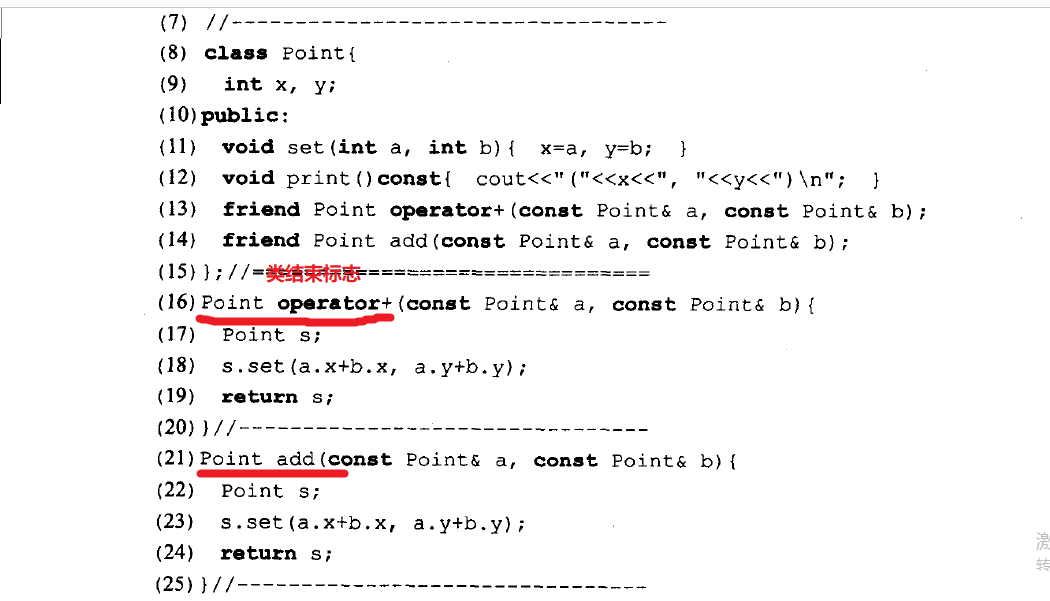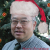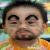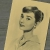## c++，这样写是不是有问题？

Point Point::operator+(const Point & a,const Point & b)
Point Point::add(const Point & a,const Point & b)21.     C++ 关键字——friend 指出："采用类的机制后实现了数据的隐藏与封装，类的数据成员一般定义为私有成员，成员函数一般定义为公有的，依此提供类与外界间的通信接口。但是，有时需要定义一些函数，这些函数不是类的一部分（注意友元函数不是类的一部分），但又需要频繁地访问类的数据成员，这时可以将这些函数定义为该函数的友元函数。"
2.     成员函数。在一个类(比如类 class Point)的定义之外 (即，花括弧之外)，书写这个类的成员函数， 必须指明是这个类 Point 的成员函数，即正如楼主所言，必须用 "Point:: + 函数名"  来标明。如果是友元 friend 函数，如楼主的案例代码，不加标注而直接书写就可以了。
3. 这里将重载操作符 + ，写成成员函数，因为这个 重载的操作符，是专门为类 Point 所用，是 Point 类的一部分。这时，就必须用到 "Point::"。

``````#include <iostream>
using namespace std;

class Point{
int x,y;
public:
Point(int ix = 0, int iy = 0){
x = ix;
y = iy;
}
void set(int a, int b){x=a;y=b;}
void print()const{  cout<< "(" <<x<< ", " <<y<<")\n"; }
//friend Point operator+(const Point& a, const Point& b);
friend Point add(const Point& a, const Point& b); //友元函数 add
Point operator+(const Point& a); //重载操作符 +
};

Point Point::operator+(const Point& a){ //类  Point 的成员函数：重载操作符 + 的定义
Point s;
s.set( x + a.x, y + a.y);
return s;
}
Point add(const Point& a, const Point& b){ //友元函数 add 的定义
Point s;
s.set(a.x+b.x, a.y+b.y);
return s;
}

int main(){
Point p0,p1(1,2),p2(3,4);
cout<<"p1: "; p1.print();
cout<<"p2: "; p2.print();
cout<<"p1 add p2: ";
p0.print();
cout<<"p1  +  p2: ";
p0=p1+p2;
p0.print();
}``````

p1: (1, 2)
p2: (3, 4)
p1 add p2: (4, 6)
p1  +  p2: (4, 6)

0Point a, b;

a + b;  //  这里的 + 号运算符的参数和书写顺序有“语法糖”转成了对人友好的写法而已；

0